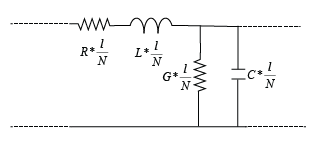# txlineLumped

Create lumped transmission line

Since R2023a

## Description

Use the new `txlineLumped` object to design lumped-L and lumped-Pi transmission lines. You can also add a `txlineLumped` element to your `rfbudget` object, `circuit` object, or in the RF Budget Analyzer app and then export the lumped transmission line to RF Blockset™.

In a lumped-L transmission line you arrange the R, L,C, and G components in an L-network topology.In a lumped-Pi transmission line, you arrange the R, L,C, and G components in a Pi-network topology.## Creation

### Syntax

``txl = txlineLumped``
``txl = txlineLumped(Name=Value)``

### Description

````txl = txlineLumped` creates a lumped transmission line object with default properties.```

example

````txl = txlineLumped(Name=Value)` sets Properties of the lumped transmission line element using one or more name-value arguments. For example, `txl = txlineLumped(R=75)` sets the resistance per unit length to `75` ohms. Properties not specified retain their default values.```

## Properties

expand all

Name of the lumped transmission line element, specified as a string scalar or a character vector.

Example: `'Lumped1'`

Data Types: `char` | `string`

Type of the lumped transmission line element, specified as one of the following:

• `'LumpedPi'` Lumped-pi transmission line element

• `'LumpedL'` Lumped-l transmission line element

Example: `'LumpedL'`

Data Types: `char` | `string`

Parameterization type to model segments in the lumped transmission line element, specified as either `'Impedance'` or `'Inductance'`.

Example: `'Inductance'`

Data Types: `char` | `string`

Resistance per unit length of the lumped transmission line, specified as a positive scalar in ohms per meter.

Example: `0.5`

Data Types: `double`

Inductance per unit length of the lumped transmission line, specified as a positive scalar in henries per meter.

Example: `2.3e-7`

#### Dependencies

To set this property, set `Parameter` to `'Inductance'`.

Data Types: `double`

Capacitance per unit length of the lumped transmission line, specified as a positive scalar in farads per meter.

Example: `6.6e-11`

Data Types: `double`

Conductance per unit length of the lumped transmission line, specified as a positive scalar in siemens per meter.

Example: `5.4e-6`

Data Types: `double`

Characteristic impedance of the lumped transmission line, specified as a positive scalar in ohms.

Example: `75`

#### Dependencies

To set this property, set `Parameter` to `'Impedance'`.

Data Types: `double`

Physical length of the lumped transmission line, specified as a positive scalar in meters.

Example: `0.0200`

Data Types: `double`

Number of input and output ports, specified as positive scalar.

Data Types: `double`

Terminals of the lumped transmission line, specified as a cell array of strings.

Data Types: `char` | `string`

## Object Functions

 `sparameters` Calculate S-parameters for RF data, network, circuit, and matching network objects `groupdelay` Group delay of S-parameter object or RF filter object or RF Toolbox circuit object `noisefigure` Calculate noise figure of transmission lines, series RLC, and shunt RLC circuits `getZ0` Calculate characteristic impedance with and without dispersion for transmission line

## Examples

collapse all

Create a lumped-L transmission line.

`txl = txlineLumped(Type='LumpedL');`

Calculate the S-parameters of the lumped-L transmission line.

`sparam = sparameters(txl,2.1e9)`
```sparam = sparameters: S-parameters object NumPorts: 2 Frequencies: 2.1000e+09 Parameters: [2x2 double] Impedance: 50 rfparam(obj,i,j) returns S-parameter Sij ```

Calculate the noise figure of the lumped-L transmission line.

`nf = noisefigure(txl,2.1e9)`
```nf = 2.1715e-05 ```

Calculate the group delay of the lumped-L transmission line.

`gd = groupdelay(txl,2.1e9)`
```gd = 4.7000e-11 ```

Calculate the characteristic impedance of the lumped-L transmission line.

`zo = getZ0(txl,2.1e9)`
```zo = 50 ```

## Version History

Introduced in R2023a